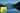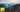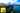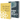# Multivariate Linear Regression, Gradient Descent in JavaScript

by Robin WieruchA recent article gave an introduction to the field of machine learning in JavaScript by in a univariate regression problem. It used plain mathematical expressions and thus made use of the unvectorized implementation of gradient descent and the cost function. However, the unvectorized approach doesn't scale when applying it for multiple parameters (e.g. ) or having a multivariate training set with multiple features n. That's the perfect point in time to use and thus to use the .

I would recommend to understand linear regression with gradient descent, the matrix operations and the implementation of vectorization first, before you continue to apply these learnings in this article in a vectorized multivariate linear regression with gradient descent. This article takes it one step further by applying the vectorized implementation of gradient descent in a multivariate instead of a univariate training set. Thus it should be possible to predict housing prices based two features: size and number of bedrooms. A linear regression with multiple variables is also known as multivariate linear regression.

I highly recommend to take the Machine Learning course by Andrew Ng. This article will not explain the machine learning algorithms in detail, but only demonstrate their usage in JavaScript. The course on the other hand goes into detail and explains these algorithms in an amazing quality. At this point in time of writing the article, I learn about the topic myself and try to internalize my learnings by writing about them and applying them in JavaScript. If you find any parts for improvements, please reach out in the comments or create a Issue/Pull Request on GitHub.

## Feature Scaling in JavaScript

Before gradient descent can be performed on the training set, it makes sense to apply feature scaling to it. The article will demonstrate it from scratch, but you will find later on the whole source code on GitHub for it. Feature scaling is one approach to . This article will demonstrate it by using a standardization as feature scaling method. Other popular feature scaling methods would be rescaling and mean normalization.

Our starting point is the following function in JavaScript whereas the other parts will be implemented while reading the article:

`import math from 'mathjs'; function init(matrix) {  let X = math.eval('matrix[:, 1:2]', {    matrix,  });  let y = math.eval('matrix[:, 3]', {    matrix,  });   let m = y.length;   // Part 1: Feature Normalization   // Part 2: Gradient Descent}`

The function signature has access to the matrix as argument which includes all the information of the training set. Each row represents one house in the training set and each column represents one feature of the house. Thus each vector in the matrix represents a feature vector. By extracting X and y from the matrix as sub matrix and vector, there is on one side the matrix X with all the features that are used for the prediction (size, number of bedrooms) and on the other side y with the outcome (price) of it. Apart from that, m represents the size of the training set (number of houses).

Now, there comes the part of the feature normalization implementation. Let's extract it as reusable function from the beginning. It should take a matrix as argument and return the normalized matrix. The normalized matrix will be used to train the hypothesis parameters by using gradient descent. Furthermore, a row vector of the feature's means and standard deviations are returned. Both are used later on to predict future housing prices when having the trained hypothesis function, because these future houses have to run through the feature scaling process too, before they can run through the trained prediction algorithm.

`function init(matrix) {   ...   // Part 1: Feature Normalization   let { XNorm, mu, sigma } = featureNormalize(X);   // Part 2: Gradient Descent} function featureNormalize(X) {  ...   return { XNorm, mu, sigma };}`

Now, since the standardization is used as feature scaling method, the function needs to calculate the mean and standard deviation of each feature vector of X and put it into a row vector.

Since I haven't found any helpful functionality in math.js to perform it, I implemented an own helper function for it. These helper functions can be found in this util library, if you don't want to implement them yourself and don't want to care about them. But for the sake of completeness, here they are:

`import math from 'mathjs'; function getMeanAsRowVector(matrix) {  const n = matrix.length;   const vectors = Array(n).fill().map((_, i) =>    math.eval(`matrix[:, \${i + 1}]`, { matrix })  );   return vectors.reduce((result, vector) =>    result.concat(math.mean(vector)), []  );} function getStdAsRowVector(matrix) {  const n = matrix.length;   const vectors = Array(n).fill().map((_, i) =>    math.eval(`matrix[:, \${i + 1}]`, { matrix })  );   return vectors.reduce((result, vector) =>    result.concat(math.std(vector)), []  );}`

Afterward, these functionalities can be used to return the mean and standard deviation of each feature as row vector.

`import {  getMeanAsRowVector,  getStdAsRowVector,} from 'mathjs-util'; ... function featureNormalize(X) {  const mu = getMeanAsRowVector(X);  const sigma = getStdAsRowVector(X);   ...   return { XNorm, mu, sigma };}`

Next, every column of matrix X needs to be normalized by using the mean and standard deviation vectors. It is possible to iterate over the features n to normalize each column (vector) of matrix X.

`function featureNormalize(X) {  const mu = getMeanAsRowVector(X);  const sigma = getStdAsRowVector(X);   const n = X.length;  for (let i = 0; i < n; i++) {    ...  }   return { XNorm, mu, sigma };}`

Now let's normalize each feature vector in matrix X. It can be done in four steps. First, extract the feature vector from it.

`function featureNormalize(X) {  const mu = getMeanAsRowVector(X);  const sigma = getStdAsRowVector(X);   const n = X.length;  for (let i = 0; i < n; i++) {    let featureVector = math.eval(`X[:, \${i + 1}]`, {      X,    });     ...  }   return { XNorm, mu, sigma };}`

Bear in mind that when using the eval method of math.js, the matrices are 1 indexed in the mathematical expression. But when you are accessing plain JavaScript matrices (arrays in array) it is 0 indexed again.

Second, subtract the mean of each value in the feature vector by using the calculated mean of the corresponding feature i.

`function featureNormalize(X) {  const mu = getMeanAsRowVector(X);  const sigma = getStdAsRowVector(X);   const n = X.length;  for (let i = 0; i < n; i++) {    let featureVector = math.eval(`X[:, \${i + 1}]`, {      X,    });     let featureMeanVector = math.eval('featureVector - mu', {      featureVector,      mu: mu[i]    });     ...  }   return { XNorm, mu, sigma };}`

Third, divide the result through the standard deviation by using the calculated standard deviation of the corresponding feature i.

`function featureNormalize(X) {  const mu = getMeanAsRowVector(X);  const sigma = getStdAsRowVector(X);   const n = X.length;  for (let i = 0; i < n; i++) {    let featureVector = math.eval(`X[:, \${i + 1}]`, {      X,    });     let featureMeanVector = math.eval('featureVector - mu', {      featureVector,      mu: mu[i]    });     let normalizedVector = math.eval('featureMeanVector / sigma', {      featureMeanVector,      sigma: sigma[i],    });     ...  }   return { XNorm, mu, sigma };}`

And fourth, replace the feature vector (column) in matrix X with the normalized vector.

`function featureNormalize(X) {  const mu = getMeanAsRowVector(X);  const sigma = getStdAsRowVector(X);   const n = X.length;  for (let i = 0; i < n; i++) {    let featureVector = math.eval(`X[:, \${i + 1}]`, {      X,    });     let featureMeanVector = math.eval('featureVector - mu', {      featureVector,      mu: mu[i]    });     let normalizedVector = math.eval('featureMeanVector / sigma', {      featureMeanVector,      sigma: sigma[i],    });     math.eval(`X[:, \${i + 1}] = normalizedVector`, {      X,      normalizedVector,    });  }   return { XNorm, mu, sigma };}`

That's it. The matrix X with all the houses is normalized now. Now the gradient descent algorithm is able to use it efficiently. In addition, the function returned the mean and standard deviation for future predictions. Check again the article about improving gradient descent regarding feature scaling to revisit this topic on a theoretical level.

## Multivariate Gradient Descent (Vectorized) in JavaScript

Now it is time to implement the gradient descent algorithm to train the theta parameters of the hypothesis function. The hypothesis function can be used later on to predict future housing prices by their number of bedrooms and size. If you recall from the about gradient descent, the algorithm takes a learning rate alpha and an initial definition of the theta parameters for the hypothesis. After an amount of iterations, it returns the trained theta parameters.

`import math from 'mathjs'; function init(matrix) {  let X = math.eval('matrix[:, 1:2]', {    matrix,  });  let y = math.eval('matrix[:, 3]', {    matrix,  });   let m = y.length;   // Part 1: Feature Normalization   let { XNorm, mu, sigma } = featureNormalize(X);   // Part 2: Gradient Descent   const ALPHA = 0.01;  const ITERATIONS = 400;   let theta = [, , ];  theta = gradientDescentMulti(XNorm, y, theta, ALPHA, ITERATIONS);} function gradientDescentMulti(X, y, theta, ALPHA, ITERATIONS) {   ...   return theta;}`

Before implementing the gradient descent algorithm in JavaScript, the normalized matrix X needs to add an intercept term. Only this way the matrix operations work for theta and X. Again, I recommend to take the machine learning course by Andrew Ng to understand the intercept term in matrix X for the vectorized implementation of gradient descent.

`function init(matrix) {   ...   // Part 2: Gradient Descent   XNorm = math.concat(math.ones([m, 1]).valueOf(), XNorm);   const ALPHA = 0.01;  const ITERATIONS = 400;   let theta = [, , ];  theta = gradientDescentMulti(XNorm, y, theta, ALPHA, ITERATIONS);}`

Now the gradient descent implementation in JavaScript. First of all, it needs to iteration over the defined iterations to train theta. Otherwise it would train it only once. Furthermore, you will need the size of the training set m for the algorithm.

`function gradientDescentMulti(X, y, theta, ALPHA, ITERATIONS) {  const m = y.length;   for (let i = 0; i < ITERATIONS; i++) {    ...  }   return theta;}`

The vectorized mathematical expression for the algorithm is straight forward. Again, the derivation of the expression can be learned in the machine learning course and partly in the referenced articles.

`theta - ALPHA / m * ((X * theta - y)' * X)'`

Since the gradient descent function has all these parameters as input in its function signature, you can simply make use of it by using the eval function of math.js.

`theta = math.eval(`theta - ALPHA / m * ((X * theta - y)' * X)'`, {  theta,  ALPHA,  m,  X,  y,});`

In the algorithm, theta would be trained with every iteration by applying gradient descent.

`function gradientDescentMulti(X, y, theta, ALPHA, ITERATIONS) {  const m = y.length;   for (let i = 0; i < ITERATIONS; i++) {    theta = math.eval(`theta - ALPHA / m * ((X * theta - y)' * X)'`, {      theta,      ALPHA,      m,      X,      y,    });  }   return theta;}`

After the defined amount of iterations, the theta vector should be trained. Finally, you can predict the price of a future house depending on the number of bedrooms and size.

`function init(matrix) {   ...   // Part 3: Predict Price of 1650 square meter and 3 bedroom house   let normalizedHouseVector = [    1,    ((1650 - mu) / sigma),    ((3 - mu) / sigma)  ];   let price = math.eval('normalizedHouseVector * theta', {    normalizedHouseVector,    theta,  });   console.log('Predicted price for a 1650 square meter and 3 bedroom house: ', price);}`

That's it. You have implemented gradient descent in JavaScript for a multivariate regression problem.

Hopefully the article helped you to understand and apply linear regression with gradient descent in a multivariate training set in JavaScript. Here you can find the whole project to try it out yourself. If you are looking for an alternative for gradient descent, check out the next article . Another article might be interesting as well, if you are keen to learn about solving .## Gradient Descent with Vectorization in JavaScript

A recent article gave an introduction to the field of machine learning in JavaScript by predicting housing prices with gradient descent in a univariate regression problem. It used plain mathematical…## Linear Regression with Normal Equation in JavaScript

A recent article gave an introduction to the field of machine learning in JavaScript by predicting housing prices with gradient descent in a univariate regression problem. It used plain mathematical…